# JavaScript Algorithms: Binary Tree Right Side View (LeetCode)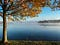Photo by Aaron Burden on Unsplash

# Description

Given a binary tree, imagine yourself standing on the right side of it, return the values of the nodes you can see ordered from top to bottom.

Example:

`Input: [1,2,3,null,5,null,4]Output: [1, 3, 4]Explanation:   1            <--- /   \2     3         <--- \     \  5     4       <---`

# Solution

Every time when you face a problem where you need to go through a tree level by level you should think about BFS — Breadth-first search.

Breadth-first search (BFS) is an algorithm for traversing or searching tree or graph data structures. It starts at the tree root (or some arbitrary node of a…

# JavaScript Algorithms: Verifying an Alien Dictionary (LeetCode)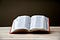Photo by Pisit Heng on Unsplash

# Description

In an alien language, surprisingly they also use English lowercase letters, but possibly in a different `order`. The `order` of the alphabet is some permutation of lowercase letters.

Given a sequence of `words` written in the alien language, and the `order` of the alphabet, return `true` if and only if the given `words` are sorted lexicographically in this alien language.

Example 1:

`Input: words = ["hello","leetcode"], order = "hlabcdefgijkmnopqrstuvwxyz"Output: trueExplanation: As 'h' comes before 'l' in this language, then the sequence is sorted.`

Example 2:

`Input: words = ["word","world","row"], order = "worldabcefghijkmnpqstuvxyz" Output: false Explanation: As 'd' comes…`

# JavaScript Algorithms: Merge intervals (LeetCode)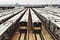Photo by Maxim Melnikov on Unsplash

# Description

Given an array of `intervals` where `intervals[i] = [starti, endi]`, merge all overlapping intervals, and return an array of the non-overlapping intervals that cover all the intervals in the input.

Example 1:

`Input: intervals = [[1,3],[2,6],[8,10],[15,18]]Output: [[1,6],[8,10],[15,18]]Explanation: Since intervals [1,3] and [2,6] overlaps, merge them into [1,6].`

Example 2:

`Input: intervals = [[1,4],[4,5]]Output: [[1,5]]Explanation: Intervals [1,4] and [4,5] are considered overlapping.`

Constraints:

• `1 <= intervals.length <= 104`
• `intervals[i].length == 2`
• `0 <= starti <= endi <= 104`

# Solution

In this problem, we can sort the `intervals` in advance and after that we can go through the sorted…

# JavaScript Algorithms: Number of Islands (LeetCode)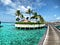Photo by Upgraded Points on Unsplash

# Description

Given an `m x n` 2d `grid` map of `'1'`s (land) and `'0'`s (water), return the number of islands.

An island is surrounded by water and is formed by connecting adjacent lands horizontally or vertically. You may assume all four edges of the grid are all surrounded by water.

Example 1:

`Input: grid = [  ["1","1","1","1","0"],  ["1","1","0","1","0"],  ["1","1","0","0","0"],  ["0","0","0","0","0"]]Output: 1`

Example 2:

`Input: grid = [  ["1","1","0","0","0"],  ["1","1","0","0","0"],  ["0","0","1","0","0"],  ["0","0","0","1","1"]]Output: 3`

Constraints:

• `m == grid.length`
• `n == grid[i].length`
• `1 <= m, n <= 300`
• `grid[i][j]` is `'0'` or `'1'`.

# Solution

Let’s take a look at Example 1.

# JavaScript Algorithms: Find All Duplicates in an Array (LeetCode)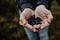Photo by Beth Jnr on Unsplash

# Description

Given an array of integers, 1 ≤ a[i] ≤ n (n = size of array), some elements appear twice and others appear once.

Find all the elements that appear twice in this array.

Could you do it without extra space and in O(n) runtime?

Example:

# Solution

The first solution that comes to my mind was two nested for loops:

# JavaScript Algorithms: Meeting Rooms (LeetCode)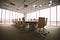Photo by Benjamin Child on Unsplash

# Description

Given an array of meeting time `intervals` where `intervals[i] = [starti, endi]`, determine if a person could attend all meetings.

Example 1:

`Input: intervals = [[0,30],[5,10],[15,20]]Output: false`

Example 2:

`Input: intervals = [[7,10],[2,4]]Output: true`

Constraints:

• `0 <= intervals.length <= 104`
• `intervals[i].length == 2`
• `0 <= starti < endi <= 106`

# Solution

I came up with a brute force solution when I had seen this question the first time. We can go through the `intervals` array and create a `Set` with all busy minutes. …

# JavaScript Algorithms: Valid Parentheses (LeetCode)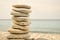Photo by Iva Rajović on Unsplash

# Description

Given a string `s` containing just the characters `'('`, `')'`, `'{'`, `'}'`, `'['` and `']'`, determine if the input string is valid.

An input string is valid if:

1. Open brackets must be closed by the same type of brackets.
2. Open brackets must be closed in the correct order.

Example 1:

`Input: s = "()"Output: true`

Example 2:

`Input: s = "()[]{}"Output: true`

Example 3:

`Input: s = "(]"Output: false`

Example 4:

`Input: s = "([)]"Output: false`

Example 5:

`Input: s = "{[]}"Output: true`

Constraints:

• `1 <= s.length <= 104`
• `s` consists of parentheses only `'()[]{}'`.

# JavaScript Algorithms: Balanced Binary Tree (LeetCode)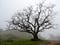Photo by Adarsh Kummur on Unsplash

# Description

Given a binary tree, determine if it is height-balanced.

For this problem, a height-balanced binary tree is defined as:

a binary tree in which the left and right subtrees of every node differ in height by no more than 1.

Example 1:

# JavaScript Algorithms: Integer to Roman (LeetCode)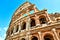Photo by Mathew Schwartz on Unsplash

# Description

Roman numerals are represented by seven different symbols: `I`, `V`, `X`, `L`, `C`, `D` and `M`.

`Symbol       ValueI             1V             5X             10L             50C             100D             500M             1000`

For example, `2` is written as `II` in Roman numeral, just two one's added together. `12` is written as `XII`, which is simply `X + II`. The number `27` is written as `XXVII`, which is `XX + V + II`.

Roman numerals are usually written largest to smallest from left to right. However, the numeral for four is not `IIII`. Instead, the number four is written as `IV`

# JavaScript Algorithms: Roman to Integer (LeetCode)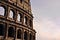Photo by Cristina Gottardi on Unsplash

# Description

Roman numerals are represented by seven different symbols: `I`, `V`, `X`, `L`, `C`, `D` and `M`.

`Symbol       ValueI             1V             5X             10L             50C             100D             500M             1000`

For example, two is written as `II` in Roman numeral, just two one's added together. Twelve is written as, `XII`which is simply `X` + `II`. The number twenty-seven is written as `XXVII`, which is `XX` + `V` + `II`.

Roman numerals are usually written largest to smallest from left to right. However, the numeral for four is not `IIII`. Instead, the number four is written as `IV`. Because…## Anatolii Kurochkin

Software Engineer | Frontend Developer | React Developer | JavaScript Developer https://anatolii.tech/